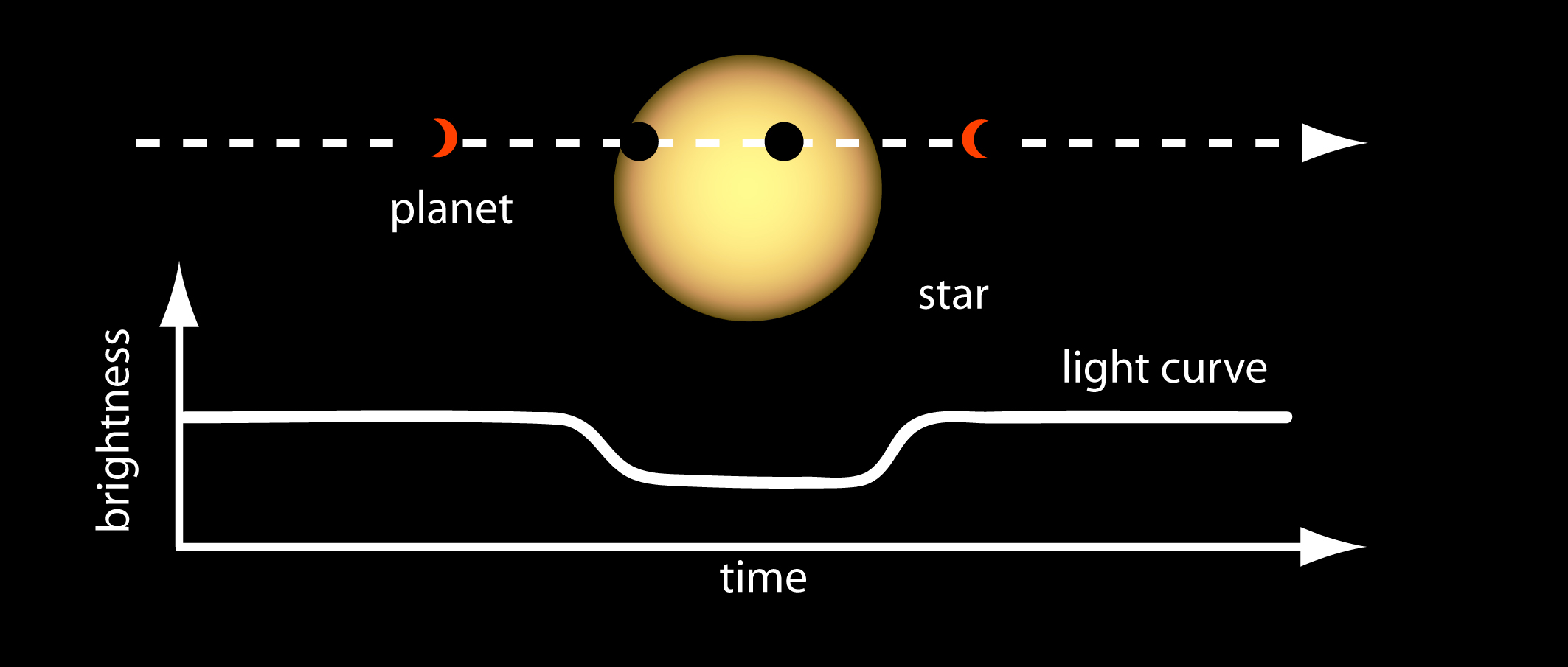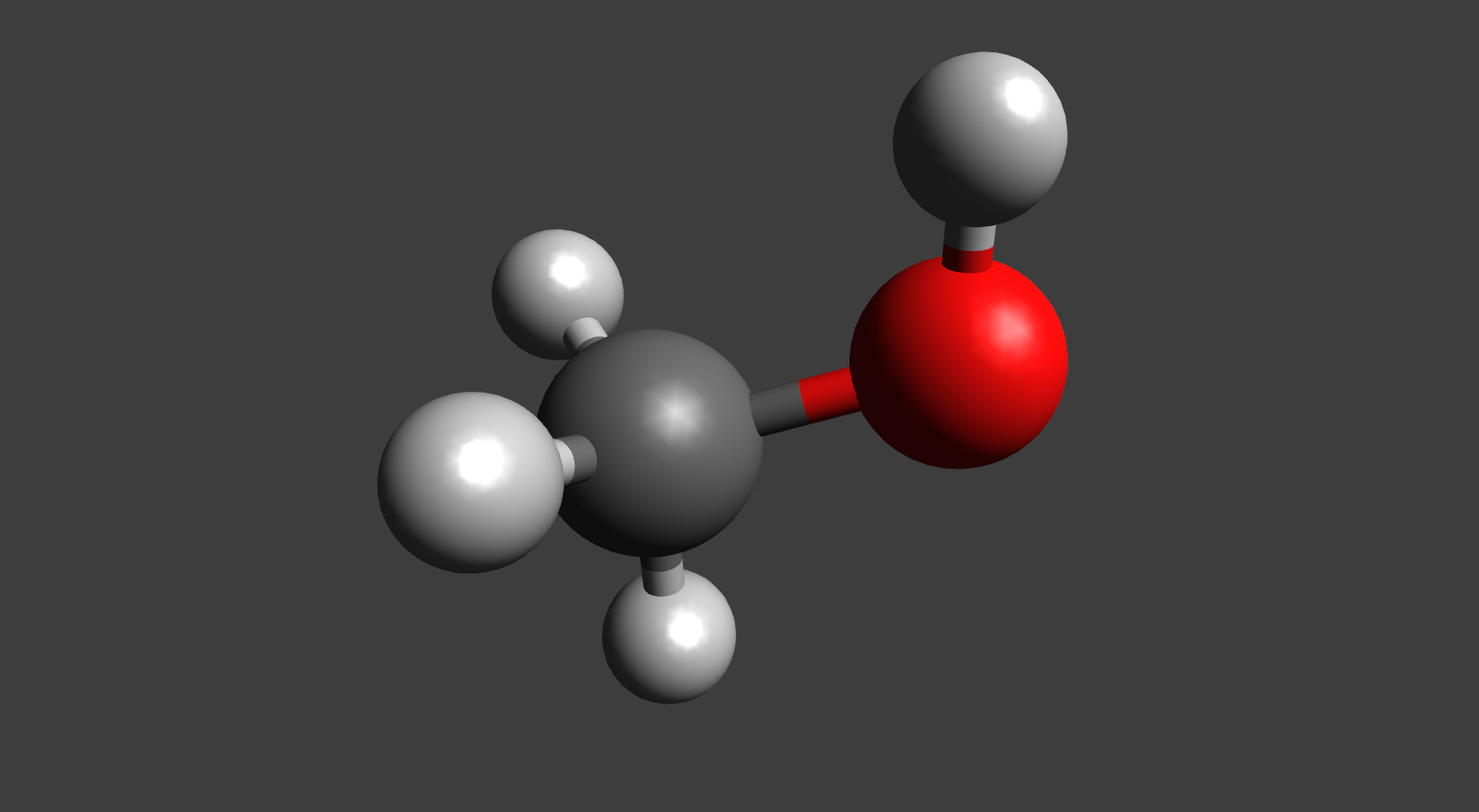## Coauthors

• Prof. Karin Öberg (Harvard)
• Prof. Ilse Cleeves (UVa)
• Prof. Leslie Rogers (UChicago)

## Outline of today's talk

1. Introduction to exoplanets
2. Project 1: SQUISHv2 and tidally distorted rocky planets
3. Introduction to planet formation
4. Project 2: Coupling accretion and chemistry in protoplanetary disks
5. Questions

# Exoplanets and their properties

## Discovering planets(source: nasa.gov)

## Detecting planets(source: jpl.nasa.gov)

## Aside: Kepler selection biases

• Large planets: deeper, stronger transit signal
• Close-in planets: more frequent transits, better identification of periodic signals

## Planets are diverse!(source: popularmechanics.com)

# Project 1: Structures and compositions of tidally-distorted rocky planets

## What are squishy planets?

• Original idea (Hachisu 1986a,b): Use potential theory and relaxation method to solve for the shapes of stars in multiple systems
• Stars modeled as polytropes, density $\propto$ pressure to some power $\gamma$
• A rocky planet will act as a fluid on geological timescales
• Use a “modified polytrope” equation of state (Seager+ 2007) $\rho = \rho_0 + A p^\gamma$

## What are squishy planets?## Methodology

1. Initialize with a guess density distribution
2. Compute the potential everywhere
3. Compute the enthalpy from the potential
4. Compute the density from the enthalpy
5. Repeat until convergence

## PySquish implementation

• Uses no internal parallelization — jobs are submitted to slurm as array jobs
• Python frontend, Cython bridge to C++ backend
• Compiled-in grid resolution
• Truncate the spherical harmonics series at a fixed number

## Results from PySquish## Results from PySquish## SQUISHv2 implementation

• Uses some pure MPI for task management
• Uses PETSc for memory management (DMDA) and ODE solve (TS)
• Compiled-in grid resolution
• Truncate the spherical harmonics series at a fixed number

## Volume render of a squishy planet# Planet formation theory

## Where do planets form?(inspired by Henning & Semenov 2013)

## Forming planets from dust

• Dust grains collide and stick, bounce, or fragment
• Grains are stickier if they have ices
• Still have barriers to formation, so not a perfect theory

## ALMA observes disks(source: theverge.com)

## ALMA observes disks(source: eso.org)

## Even more substructure in disks(source: almascience.eso.org, see Andrews, Huang+ 2018 and associated papers)

## Observed early planets(source: nature.com, Haffert+ 2019)

## Formation environment is important(inspired by Henning & Semenov 2013)

## Disk chemistryFormaldehydeMethanolHydrogen cyanide

## Disk chemistry(courtesy of Karin Öberg)

# Project 2: Coupling chemistry and accretion in a protoplanetary disk

## Problem statement

We want to know how accretion affects the chemistry that occurs in disks to gain insight into the compositions of planets that can form from the disk material.

## Global solution

• Can do a single, “global” simulation of disk chemistry
• Pros:
• Can incorporate as much physics as needed
• Cons:
• Very computationally expensive!
• If we have $M$ species and $N$ computational cells and cells are not independent, would need a $M N \times M N$ (sparse) matrix to solve for all chemistry at a single timestep
• Examples given in Haworth+ (2016)

## Alternative: reduce chemical network

• Can choose a subset of “important” reactions to reduce number of reactions and species being considered
• Pros:
• Can incorporate as much physics as needed
• Cons:
• Lose chemical insight
• Chemical network reduction is a science/art itself!

## Alternative: reduce physics

• Can reduce the physical processes considered
• Common approach is to assume that disk material is not moving radially or vertically
• Pros:
• No loss of chemical complexity
• Cons:
• Missing (possibly) important effects of dynamic processes

## Alternative: local simulation

• Solve for accretion tracks self-consistently and follow chemistry along tracks
• Pros:
• Under some assumptions, no compromise between chemistry and physics
• Approximately equal computational load to a static model
• Can be built upon in future work
• Example: Heinzeller, Nomura, & Walsh (2011)

## Surface density

$$\frac{\partial \Sigma}{\partial t} - \frac{3}{R} \frac{\partial}{\partial R} \left[R^{1/2} \frac{\partial}{\partial R} \left(\nu \Sigma R^{1/2}\right)\right] = 0$$
• From Lynden-Bell & Pringle (1974) and Pringle (1981)
• By combining the Navier-Stokes and mass continuity equation, we find a diffusion-type equation in surface density $\Sigma$

## Chemistry post-processing

Consider chemistry in a “box”

$$n_i + n_j \rightarrow \cdots$$ $$n_{j1} + n_{j2} \rightarrow n_i + \cdots$$

Chemical network: reactions with rates $P_j$ and $R_j$

$$\frac{\mathrm{d} n_i}{\mathrm{d} t}\bigg|_V = \sum\limits_j P_j n_{j1} n_{j2} - n_i \sum\limits_j R_j n_j$$

## Tracks through the disk(source: Price, Cleeves, & Öberg, 2019, in prep.)

## Does accretion affect chemistry?(source: Price, Cleeves, & Öberg, 2019, in prep.)

## Acknowledgements

• Used in this presentation:
• Used in the projects: